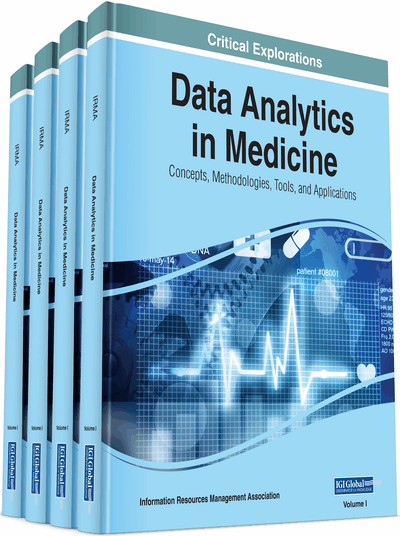# Relationships between Models of Genetic Regulatory Networks with Emphasis on Discrete State Stochastic Models

Randip Pal (Texas Tech University, USA)
DOI: 10.4018/978-1-7998-1204-3.ch012

## Abstract

Genetic Regulatory Networks (GRNs) represent the interconnections between genomic entities that govern the regulation of gene expression. GRNs have been represented by various types of mathematical models that capture different aspects of the biological system. This chapter discusses the relationships among the most commonly used GRN models that can enable effective integration of diverse types of sub-models. A detailed model in the form of stochastic master equation is described, followed by it coarse-scale and deterministic approximations in the form of Probabilistic Boolean Networks and Ordinary Differential Equation models respectively.
Chapter Preview
Top

## Introduction

Genetic Regulatory Networks (GRNs) represent the interconnections between genomic entities that govern the regulation of gene expression. Since biological regulatory networks are extremely detailed with numerous interactions, a single mathematical model to represent the whole biological regulatory system is generally not feasible. Depending on the purpose of modeling, the mathematical model representing the GRN brings in a level of abstraction. The focus of the modeling can be capturing interactions between RNA expressions, protein-protein interactions or interactions between metabolites. Usually, only parts of the regulome (i.e. genes, proteins and metabolites involved in gene regulation) such as transcription factors, enhancers, microRNA etc. are made explicit in a mathematical model of a GRN.

To provide further background on genetic regulatory network modeling, we present broad classifications of the models that are commonly used to capture the relationships between genomic entities. GRN models usually belong to either static or dynamical models. Static models are concerned with providing the topology of the network (such as the connectivity of various genomic entities) without attempting to model the dynamics of the interactions. Dynamic models on the other hand not only capture the connectivity of the network entities but also provide the time dependent functional relationships that govern the interactions. Commonly used pathway models as presented in databases such as KEGG and StringDB belong to the category of static models as they primarily capture the connectivity structure without the network dynamics. Examples of dynamic models include ordinary differential equation models to model the average concentration of mRNA expressions (Kampen, 1991) or Dynamic Bayesian Network (Murphy, 2002) models. Based on the model complexity involving inference of connectivity and time dependent relationships, the experimental data requirements for elucidating dynamic models are significantly higher as compared to static models.

To further explore various levels of time dependent behavior of genes or proteins, dynamic network models can be essentially categorized as discrete where the amount of genomic entity is modeled as discrete values or continuous where the genomic entities are models as continuous variables. Since the dynamics of biological entities consist of discrete changes based on the number of molecules created or destroyed, a fine-scale discrete model can potentially faithfully capture the dynamics of the genomic entities. Note that we have categorized the dynamic models as discrete or continuous based on the representation of the genomic entity but a similar categorization can be applied based on discrete or continuous representation of time. Some models such as stochastic master equations (also commonly known as Chemical Master Equations) incorporate time as a continuous quantity whereas models like Boolean Networks update states at discrete time intervals. The next level of characterizations is based on whether the models considered are stochastic or deterministic. A number of studies have shown that the generation of mRNA or protein expression is stochastic in nature (Arkin, Ross, & McAdams, 1998) and thus a stochastic model is suitable to capture the true change in state probability distribution with time. However, available experimental data may limit the inference of the parameters of a stochastic model. For instance, common genomic characterizations using microarrays provide an average observation of the mRNA expressions and are not suitable for inferring the parameters of a detailed stochastic model.

## Complete Chapter List

Search this Book:
Reset